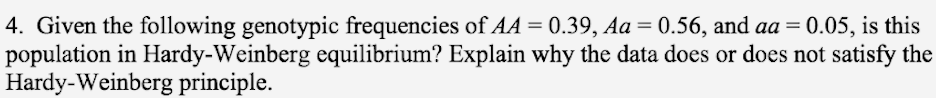# Problem: Given the following genotypic frequencies of AA = 0.39, Aa = 0.56, and aa = 0.05, is this population in Hardy-Weinberg equilibrium? Explain why the data does or does not satisfy the Hardy-Weinberg equilibrium.

###### FREE Expert Solution

In checking if a population follows the Hardy-Weinberg equilibrium for a certain trait, the sum of the relative frequency of p (dominant allele) and q (recessive allele) should be equal to 1.

p2 + 2pq + q2 = 1

p + q = 1###### Problem Details

Given the following genotypic frequencies of AA = 0.39, Aa = 0.56, and aa = 0.05, is this population in Hardy-Weinberg equilibrium? Explain why the data does or does not satisfy the Hardy-Weinberg equilibrium.